sbioparameterci

Compute confidence intervals for estimated parameters (requires Statistics and Machine Learning Toolbox)

Description

example

ci = sbioparameterci(fitResults) computes 95% confidence intervals for the estimated parameters from fitResults, an NLINResults object or OptimResults object returned by the sbiofit function. ci is a ParameterConfidenceInterval object that contains the computed confidence intervals.

example

ci = sbioparameterci(fitResults,Name,Value) uses additional options specified by one or more Name,Value pair arguments.

Examples

collapse all

Load the sample data to fit. The data is stored as a table with variables ID , Time , CentralConc , and PeripheralConc. This synthetic data represents the time course of plasma concentrations measured at eight different time points for both central and peripheral compartments after an infusion dose for three individuals.

gData = groupedData(data);
gData.Properties.VariableUnits = {'','hour','milligram/liter','milligram/liter'};
sbiotrellis(gData,'ID','Time',{'CentralConc','PeripheralConc'},'Marker','+',...
'LineStyle','none');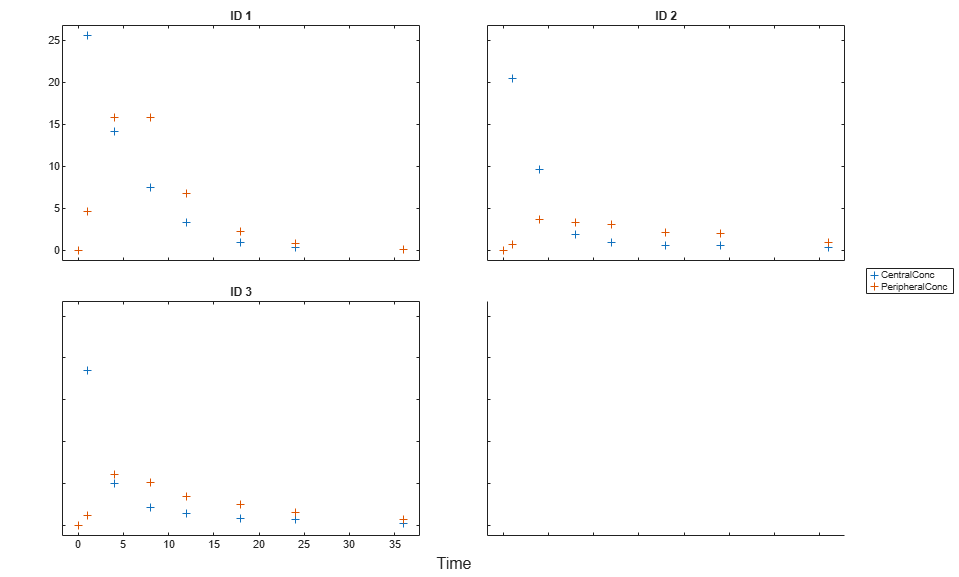Create Model

Create a two-compartment model.

pkmd                 = PKModelDesign;
pkc1.DosingType      = 'Infusion';
pkc1.EliminationType = 'linear-clearance';
pkc1.HasResponseVariable = true;
model                = construct(pkmd);
configset            = getconfigset(model);
configset.CompileOptions.UnitConversion = true;

Define Dosing

Define the infusion dose.

dose             = sbiodose('dose','TargetName','Drug_Central');
dose.StartTime   = 0;
dose.Amount      = 100;
dose.Rate        = 50;
dose.AmountUnits = 'milligram';
dose.TimeUnits   = 'hour';
dose.RateUnits   = 'milligram/hour';

Define Parameters

Define the parameters to estimate. Set the parameter bounds for each parameter. In addition to these explicit bounds, the parameter transformations (such as log, logit, or probit) impose implicit bounds.

responseMap = {'Drug_Central = CentralConc','Drug_Peripheral = PeripheralConc'};
paramsToEstimate   = {'log(Central)','log(Peripheral)','Q12','Cl_Central'};
estimatedParam     = estimatedInfo(paramsToEstimate,...
'InitialValue',[1 1 1 1],...
'Bounds',[0.1 3;0.1 10;0 10;0.1 2]);

Fit Model

Perform an unpooled fit, that is, one set of estimated parameters for each patient.

unpooledFit = sbiofit(model,gData,responseMap,estimatedParam,dose,'Pooled',false);

Perform a pooled fit, that is, one set of estimated parameters for all patients.

pooledFit = sbiofit(model,gData,responseMap,estimatedParam,dose,'Pooled',true);

Compute Confidence Intervals for Estimated Parameters

Compute 95% confidence intervals for each estimated parameter in the unpooled fit.

ciParamUnpooled = sbioparameterci(unpooledFit);

Display Results

Display the confidence intervals in a table format. For details about the meaning of each estimation status, see Parameter Confidence Interval Estimation Status.

ci2table(ciParamUnpooled)
ans =

12x7 table

Group         Name         Estimate    ConfidenceInterval      Type      Alpha      Status
_____    ______________    ________    __________________    ________    _____    ___________

1      {'Central'   }      1.422      1.1533     1.6906    Gaussian    0.05     estimable
1      {'Peripheral'}     1.5629     0.83143     2.3551    Gaussian    0.05     constrained
1      {'Q12'       }    0.47159     0.20093    0.80247    Gaussian    0.05     constrained
1      {'Cl_Central'}    0.52898     0.44842    0.60955    Gaussian    0.05     estimable
2      {'Central'   }     1.8322      1.7893     1.8751    Gaussian    0.05     success
2      {'Peripheral'}     5.3368      3.9133     6.7602    Gaussian    0.05     success
2      {'Q12'       }    0.27641      0.2093    0.34351    Gaussian    0.05     success
2      {'Cl_Central'}    0.86034     0.80313    0.91755    Gaussian    0.05     success
3      {'Central'   }     1.6657      1.5818     1.7497    Gaussian    0.05     success
3      {'Peripheral'}     5.5632      4.7557     6.3708    Gaussian    0.05     success
3      {'Q12'       }    0.78361     0.65581    0.91142    Gaussian    0.05     success
3      {'Cl_Central'}     1.0233     0.96375     1.0828    Gaussian    0.05     success

Plot the confidence intervals. If the estimation status of a confidence interval is success, it is plotted in blue (the first default color). Otherwise, it is plotted in red (the second default color), which indicates that further investigation into the fitted parameters may be required. If the confidence interval is not estimable, then the function plots a red line with a centered cross. If there are any transformed parameters with estimated values 0 (for the log transform) and 1 or 0 (for the probit or logit transform), then no confidence intervals are plotted for those parameter estimates. To see the color order, type get(groot,'defaultAxesColorOrder').

Groups are displayed from left to right in the same order that they appear in the GroupNames property of the object, which is used to label the x-axis. The y-labels are the transformed parameter names.

plot(ciParamUnpooled)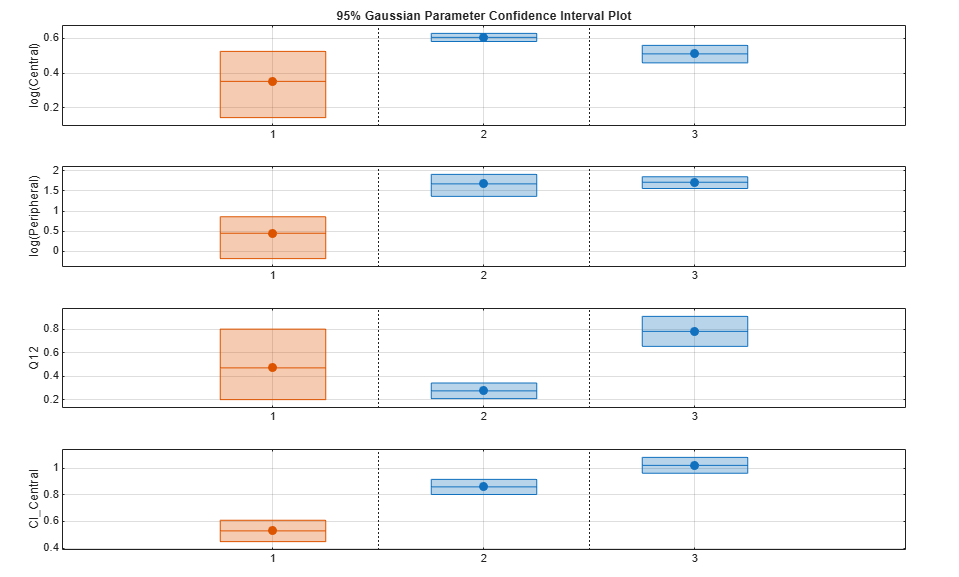Compute the confidence intervals for the pooled fit.

ciParamPooled = sbioparameterci(pooledFit);

Display the confidence intervals.

ci2table(ciParamPooled)
ans =

4x7 table

Group          Name         Estimate    ConfidenceInterval      Type      Alpha      Status
______    ______________    ________    __________________    ________    _____    ___________

pooled    {'Central'   }     1.6626      1.3287     1.9965    Gaussian    0.05     estimable
pooled    {'Peripheral'}      2.687     0.89848     4.8323    Gaussian    0.05     constrained
pooled    {'Q12'       }    0.44956     0.11445    0.85152    Gaussian    0.05     constrained
pooled    {'Cl_Central'}    0.78493     0.59222    0.97764    Gaussian    0.05     estimable

Plot the confidence intervals. The group name is labeled as "pooled" to indicate such fit.

plot(ciParamPooled)Plot all the confidence interval results together. By default, the confidence interval for each parameter estimate is plotted on a separate axes. Vertical lines group confidence intervals of parameter estimates that were computed in a common fit.

ciAll = [ciParamUnpooled;ciParamPooled];
plot(ciAll)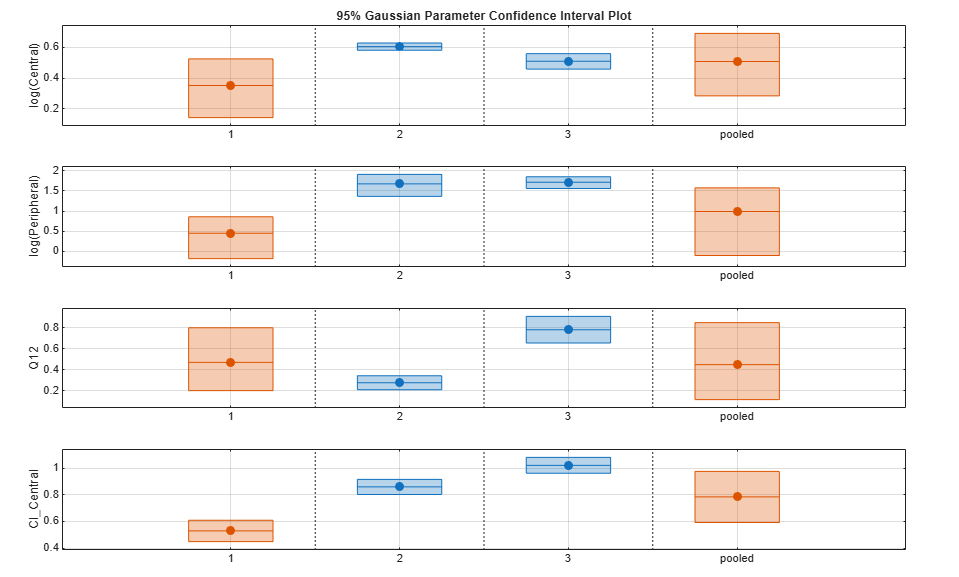You can also plot all confidence intervals in one axes grouped by parameter estimates using the 'Grouped' layout.

plot(ciAll,'Layout','Grouped')In this layout, you can point to the center marker of each confidence interval to see the group name. Each estimated parameter is separated by a vertical black line. Vertical dotted lines group confidence intervals of parameter estimates that were computed in a common fit. Parameter bounds defined in the original fit are marked by square brackets. Note the different scales on the y-axis due to parameter transformations. For instance, the y-axis of Q12 is in the linear scale, but that of Central is in the log scale due to its log transform.

Compute Confidence Intervals for Model Predictions

Calculate 95% confidence intervals for the model predictions, that is, simulation results using the estimated parameters.

% For the pooled fit
ciPredPooled = sbiopredictionci(pooledFit);
% For the unpooled fit
ciPredUnpooled = sbiopredictionci(unpooledFit);

Plot Confidence Intervals for Model Predictions

The confidence interval for each group is plotted in a separate column, and each response is plotted in a separate row. Confidence intervals limited by the bounds are plotted in red. Confidence intervals not limited by the bounds are plotted in blue.

plot(ciPredPooled)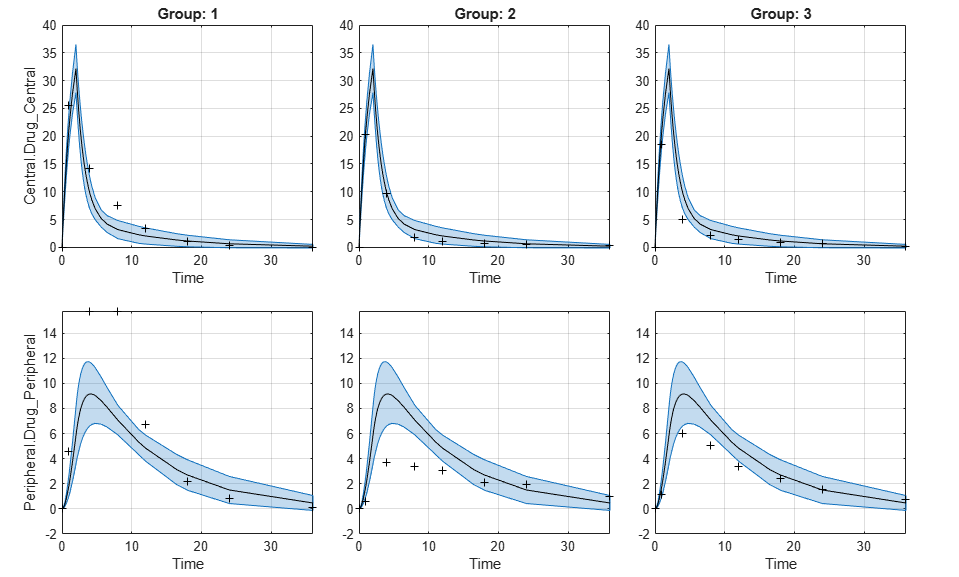plot(ciPredUnpooled)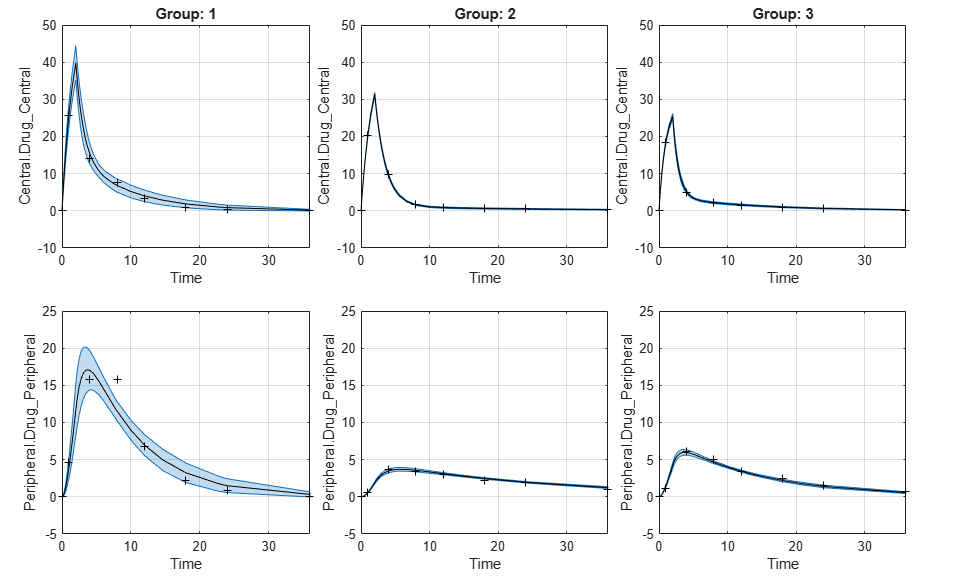Input Arguments

collapse all

Parameter estimation results from sbiofit, specified as an NLINResults object, OptimResults object, or a vector of objects for unpooled fits that were returned from the same sbiofit call.

Name-Value Arguments

Specify optional comma-separated pairs of Name,Value arguments. Name is the argument name and Value is the corresponding value. Name must appear inside quotes. You can specify several name and value pair arguments in any order as Name1,Value1,...,NameN,ValueN.

Example: 'Alpha',0.01,'Type','profileLikelihood' specifies to compute a 99% confidence interval using the profile likelihood approach.

Depending on the type of confidence interval, the compatible name-value arguments differ. The table below lists all the name-value arguments and their corresponding confidence interval types. A check mark (✔) indicates that the name-value argument is applicable for that type.

Name-Value ArgumentGaussian (default)Optimization-based profile likelihoodIntegration-based profile likelihoodBootstrap
Alpha
Type
Display
UseParallel
NumSamples
Tolerance
Parameters
MaxStepSize
UseIntegration
IntegrationOptions

Confidence level, (1-Alpha) * 100%, specified as the comma-separated pair consisting of 'Alpha' and a positive scalar between 0 and 1. The default value is 0.05, meaning a 95% confidence interval is computed.

Example: 'Alpha',0.01

Confidence interval type, specified as the comma-separated pair consisting of 'Type' and a character vector. The valid choices are:

• 'gaussian' — Use the Gaussian approximation of the distribution of parameter estimates.

• 'profileLikelihood' — Compute the profile likelihood intervals. The function has two methods to compute profile likelihood curves. By default, the function uses the optimization-based method. To use the integration-based method, you must also set 'UseIntegration' to true.

The optimization-based method fixes one parameter value at a time and reruns an optimization to compute the maximum likelihood. This optimization is done for every parameter and every point on the curve of the profile likelihood. The integration-based method is based on integrating the differential equations derived from the Lagrange equations of the optimization-based method. For details about these two methods, see Profile Likelihood Confidence Interval Calculation.

Note

This type is not supported for parameter estimates from hierarchical models, that is, estimated results from fitting different categories (such as age or sex). In other words, if you set the CategoryVariableName property of the EstimatedInfo object in your original fit, then the fit results are hierarchical and you cannot compute the profileLikelihood confidence intervals on the results.

• 'bootstrap' — Compute confidence intervals using the bootstrap method.

Example: 'Type','bootstrap'

Level of display returned to the command line, specified as the comma-separated pair consisting of 'Display' and a character vector. 'off' (default) or 'none' displays no output. 'final' displays a message when a computation finishes. 'iter' displays output at each iteration.

Example: 'Display','final'

Logical flag to compute confidence intervals in parallel, specified as the comma-separated pair consisting of 'UseParallel' and true or false. By default, the parallel options in the original fit are used. If this argument is set to true and Parallel Computing Toolbox™ is available, the parallel options in the original fit are ignored, and confidence intervals are computed in parallel.

For the Gaussian confidence intervals:

• If the input fitResults is a vector of results objects, then the computation of confidence intervals for each object is performed in parallel. The Gaussian confidence intervals are quick to compute. So, it might be more beneficial to parallelize the original fit (sbiofit) and not set UseParallel to true for sbioparameterci.

For the Profile Likelihood confidence intervals:

• If the number of results objects in the input fitResults vector is greater than the number of estimated parameters, then the computation of confidence intervals for each object is performed in parallel.

• Otherwise, the confidence intervals for all estimated parameters within one results object are computed in parallel before the function moves on to the next results object.

For the Bootstrap confidence intervals:

• The function forwards the UseParallel flag to bootci. There is no parallelization over the input vector of results objects.

Note

If you have a global stream for random number generation with several substreams to compute in parallel in a reproducible fashion, sbioparameterci first checks to see if the number of workers is same as the number of substreams. If so, sbioparameterci sets UseSubstreams to true in the statset option and passes it to bootci (Statistics and Machine Learning Toolbox). Otherwise, the substreams are ignored by default.

Example: 'UseParallel',true

Number of samples for bootstrapping, specified as the comma-separated pair consisting of 'NumSamples' and a positive integer. This number defines the number of fits that are performed during the confidence interval computation to generate bootstrap samples. The smaller the number is, the faster the computation of the confidence intervals becomes, at the cost of decreased accuracy.

Example: 'NumSamples',500

Tolerance for the profile likelihood and bootstrap confidence interval computations, specified as the comma-separated pair consisting of 'Tolerance' and a positive scalar.

The profile likelihood method uses this value as a termination tolerance. For details, see Profile Likelihood Confidence Interval Calculation.

The bootstrap method uses this value to determine whether a confidence interval is constrained by bounds specified in the original fit. For details, see Bootstrap Confidence Interval Calculation.

Example: 'Tolerance',1e-6

Names of parameters for which the profile likelihood curves are calculated, specified as a character vector, string, string vector, or cell array of character vectors. By default, the function computes the confidence intervals for all parameters listed in the Property Summary property of the fitResults object. You can also specify a subset of those parameters if needed.

Note

This name-value argument is applicable only when you specify Type as 'profileLikelihood'.

Example: 'Parameters',{'ka'}

Maximum step size used for computing profile likelihood curves, specified as the comma-separated pair consisting of 'MaxStepSize' and a positive scalar, [], or cell array.

• For the optimization-based method, the default value is 0.1. If you set 'MaxStepSize' to [], then the maximum step size is set to 10% of the width of the Gaussian approximation of the confidence interval, if it exists. You can specify a maximum step size (or []) for each estimated parameter using a cell array.

• For the integration-based method, the default value is Inf. Internally, the function uses the ode15s solver.

Example: 'MaxStepSize',0.5

Flag to use the integration-based profile likelihood confidence interval method, specified as true or false. The integration-based method integrates differential equations derived from the Lagrange equations. By default, the function uses the optimization-based method. For details about these two methods, see Profile Likelihood Confidence Interval Calculation.

Example: 'UseIntegration',true

Options for the integration-based profile likelihood confidence interval method, specified as a structure. Specify options as fields of the structure as follows.

Field NameField Value Description
Hessian

'finiteDifference' — Use the finite difference approximation of the Hessian matrix. This is the default value.

'identity' — Use the identity matrix as the Hessian matrix approximation. You must also specify a positive CorrectionFactor value.

CorrectionFactorNonnegative scalar. The default value is 0.
AbsoluteTolerancePositive scalar for the step size control in ode15s. The default value is 1e-2.
RelativeTolerancePositive scalar less than 1 for the step size control in ode15s. The default value is 1e-2.
InitialStepSizePositive scalar as the initial step size for solving the differential equations. If a parameter is bounded, the function uses the default initial step size of ode15s. If not, it uses 1e-4.

Output Arguments

collapse all

Confidence interval results, returned as a ParameterConfidenceInterval object. For an unpooled fit, ci can be a vector of ParameterConfidenceInterval objects.

collapse all

Gaussian Confidence Interval Calculation

The function uses the Wald test statistic  to compute the confidence intervals. Assuming that there are enough data, the parameter estimates, Pest, are approximately Student's t-distributed with the covariance matrix S (the CovarianceMatrix property of the results object) returned by sbiofit.

The confidence interval for the ith parameter estimate Pest,i is computed as follows:

$Pest,i±\sqrt{Si,i}*Tinv\left(1-\frac{Alpha}{2}\right)$, where Tinv is the Student's t inverse cumulative distribution function (tinv (Statistics and Machine Learning Toolbox)) with the probability 1-(Alpha/2), and Si,i is the diagonal element (variance) of the covariance matrix S.

In cases where the confidence interval is constrained by the parameter bounds defined in the original fit, the confidence interval bounds are adjusted according to the approach described by Wu, H. and Neale, M. .

Setting Estimation Status

• For each parameter estimate, the function first decides whether the confidence interval of the parameter estimate is unbounded. If so, the function sets the estimation status of the corresponding parameter estimate to not estimable.

• Otherwise, if the confidence interval for a parameter estimate is constrained by a parameter bound defined in the original fit, the function sets the estimation status to constrained. Parameter transformations (such as log, probit, or logit) impose implicit bounds on the estimated parameters, for example, positivity constraints. Such bounds can lead to the overestimation of confidence, that is, the confidence interval can be smaller than expected.

• If no confidence interval has the estimation status not estimable or constrained, then the function sets the estimation statuses of all parameter estimates to success. Otherwise, the estimation statuses of remaining parameter estimates are set to estimable.

Profile Likelihood Confidence Interval Calculation

Define L to be the likelihood, LH, of the parameter estimates (stored in the ParameterEstimates property of the results object) returned by sbiofit, $L=LH\left(Pest\right)$, where Pest is a vector of parameter estimates, Pest,1, Pest,2, …, Pest,n.

The profile likelihood function PL for a parameter Pi is defined as $PL\left(Pi\right)=\underset{Pj,j\ne i}{\mathrm{max}}LH\left(P1,...,Pi,..,Pn\right)$, where n is the total number of parameters.

Per Wilks's Theorem , the likelihood ratio test statistic, $-2\mathrm{log}\left(\frac{PL\left(Pi\right)}{L}\right)$, is chi-square distributed with 1 degree of freedom.

Therefore, find all Pi so that: $\mathrm{log}\left(L\right)-\mathrm{log}\left(PL\left({P}_{i}\right)\right)\le \frac{chiinv\left(1,1-alpha\right)}{2}$.

Equivalently, $\mathrm{log}\left(PL\left({P}_{i}\right)\right)\ge \mathrm{log}\left(L\right)-\frac{chiinv\left(1,1-alpha\right)}{2}$, where $\mathrm{log}\left(L\right)-\frac{chiinv\left(1,1-alpha\right)}{2}$ is the target value used in computing the log profile likelihood curve. The function provides two methods to compute such curve.

Optimization-based Method to Compute Log Profile Likelihood Curve

1. Start at Pest,i and evaluate the likelihood L.

2. Compute the log profile likelihood at Pest,i + k * MaxStepSize for each side (or direction) of the confidence interval, that is, k = 1, 2, 3,… and k = -1, -2, -3,….

3. Stop if one of these stopping criteria is met on each side.

• The log profile likelihood falls below the target value. In this case, start bisecting between Pbelow and Pabove, where Pbelow is the parameter value with the largest log profile likelihood value below the target value, and Pabove the parameter value with the smallest log profile likelihood value greater than the target value. Stop the bisection if one of the following is true:

• Either neighboring log profile likelihood values are less than Tolerance apart. Set the status for the corresponding side of the confidence interval to success.

• The bisection interval becomes smaller than max(Tolerance,2*eps('double')) and the profile likelihood curve computed so far is above the target value. Set the status of the corresponding side to not estimable.

• The linear gradient approximation of the profile likelihood curve (finite difference between two neighboring parameter values) is larger than -Tolerance (the negative value of the tolerance). Set the status of the corresponding side to not estimable.

• The step is limited by a bound defined in the original fit. Evaluate at the bound and set the status of the corresponding side to constrained.

Integration-Based Method to Compute Log Profile Likelihood Curve

This method  solves the constrained optimization problem $PL\left(Pi\right)=\underset{Pj,j\ne i}{\mathrm{max}}LH\left(P1,...,Pi,..,Pn\right)$ by integrating the differential equations derived from the Lagrange equations

$\begin{array}{c}-{\nabla }_{\stackrel{\to }{p}}L\left(\stackrel{\to }{p}\left(c\right)\right)+\lambda \left(c\right){\stackrel{\to }{e}}_{i}=0\text{\hspace{0.17em}}\text{\hspace{0.17em}}\\ \stackrel{\to }{p}{\left(}_{c}=c\end{array}$

Here, ${\stackrel{\to }{e}}_{i}$ is the ith canonical unit vector, the Lagrange multiplier is $\lambda \left(c\right)$, and c = Pi.

In other words, instead of optimizing point by point, this method solves differential equations that define the profile likelihood curve as follows.

$\left(\begin{array}{cc}-{\nabla }_{\stackrel{\to }{p}}^{2}L\left(\stackrel{\to }{p}\left(c\right)\right)& {\stackrel{\to }{e}}_{i}\\ ±{\stackrel{\to }{e}}_{i}^{T}& 0\end{array}\right)\left(\begin{array}{c}\stackrel{˙}{\stackrel{\to }{p}}\left(c\right)\\ \stackrel{˙}{\lambda }\left(c\right)\end{array}\right)=\left(\begin{array}{c}0\\ 1\end{array}\right)$

Here, $\stackrel{˙}{\stackrel{\to }{p}}\left(c\right)=\frac{\partial \stackrel{\to }{p}\left(c\right)}{\partial c},\stackrel{˙}{\lambda }\left(c\right)=\frac{\partial \lambda \left(c\right)}{\partial c},and-{\nabla }_{\stackrel{\to }{p}}^{2}L\left(\stackrel{\to }{p}\left(c\right)\right)$ is the Hessian of the log likelihood function.

Using the finite-difference approximation of the Hessian matrix is recommended. However, the numerical computation of the Hessian matrix using finite differencing can be computationally expensive. To reduce the computational costs, Chen and Jennrich  proposed an approximate version based on the assumption that the second-order sufficient Karush-Kuhn-Tucker conditions must hold with strict inequality at every point in the domain of the profile likelihood curve as outlined in Assumption 2 in the Appendix of . In other words, at every point on the profile likelihood curve, the remaining parameters must be estimable.

If this assumption holds, then the Hessian can be replaced with the identity matrix I as follows:

$\left(\begin{array}{cc}-I& {\stackrel{\to }{e}}_{i}\\ ±{\stackrel{\to }{e}}_{i}^{T}& 0\end{array}\right)\left(\begin{array}{c}\stackrel{˙}{\stackrel{\to }{p}}\left(c\right)\\ \stackrel{˙}{\lambda }\left(c\right)\end{array}\right)=\left(\begin{array}{c}-\gamma {\nabla }_{\stackrel{\to }{p}}L\left(\stackrel{\to }{p}\left(c\right)\right)\\ 1\end{array}\right)$

Here, ${\nabla }_{\stackrel{\to }{p}}L\left(\stackrel{\to }{p}\left(c\right)\right)$ is the gradient of the log likelihood and γ is a correction factor to ensure the solution of the differential equation stays on the path of the profile likelihood curve.

If γ is too small, the approximation of the profile likelihood curve may become inaccurate, resulting in an underestimation of the profile likelihood confidence intervals. Setting γ to a large value ensures accurate results, but might require ode15s to take smaller steps, which increases the computational cost.

Tip

You can specify the Hessian approximation and correction factor using the IntegrationOptions name-value argument.

The stopping criterion of the algorithm is when one of the following conditions becomes true:

• The gradient approximation of the profile likelihood curve is larger than -Tolerance.

• The profile likelihood falls below the target value.

• A parameter bound is reached.

Setting Estimation Status

• If both sides of the confidence interval are unsuccessful, that is, have the status not estimable, the function sets the estimation status (ci.Results.Status) to not estimable.

• If no side has the status not estimable and one side has the status constrained, the function sets the estimation status (ci.Results.Status) to constrained.

• If the computation for all parameters on both sides of the confidence intervals is successful, set the estimation status (ci.Results.Status) to success.

• Otherwise, the function sets the estimation statuses of the remaining parameter estimates to estimable.

Bootstrap Confidence Interval Calculation

The bootci (Statistics and Machine Learning Toolbox) function from Statistics and Machine Learning Toolbox™ is used to compute the bootstrap confidence intervals. The first input nboot is the number of samples (NumSamples), and the second input bootfun is a function that performs these actions:

• Resample the data (independently within each group, if multiple groups are available).

• Run a parameter fit with the resampled data.

• Return the estimated parameters.

Setting Estimation Status

If a confidence interval is closer than Tolerance to a parameter bound, as defined in the original fit, the function sets the estimation status to constrained. If all confidence intervals are further away from the parameter bounds than Tolerance, the function sets the status to success. Otherwise, it is set to estimable.

 Wald, A. "Tests of Statistical Hypotheses Concerning Several Parameters when the Number of Observations is Large." Transactions of the American Mathematical Society. 54 (3), 1943, pp. 426-482.

 Wu, H., and M.C. Neale. "Adjusted Confidence Intervals for a Bounded Parameter." Behavior Genetics. 42 (6), 2012, pp. 886-898.

 Wilks, S.S. "The Large-Sample Distribution of the Likelihood Ratio for Testing Composite Hypotheses." The Annals of Mathematical Statistics. 9 (1), 1938, pp. 60–62.

 Chen, Jian-Shen, and Robert I. Jennrich. “Simple Accurate Approximation of Likelihood Profiles.” Journal of Computational and Graphical Statistics 11, no. 3 (September 2002): 714–32.

SimBiology DocumentationGet trial now